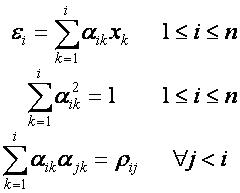### Option Pricing - Generating Correlated Random Sequences

Monte-Carlo methods are ideal for option pricing where the payoff is dependent on a basket of underlying assets, such as a spread option. However generating and using independent random paths for each asset will result in simulation paths that do not reflect how the assets in the basket have historically been correlated.

This option pricing tutorial discusses how to generate sequences of correlated random numbers so that when used to price an option on a basket of assets the simulation paths reflect the historical correlation between the assets.

For a discussion of the basic mathematics underlying Monte-Carlo simulation as used in option pricing see the Monte-Carlo tutorial. An example of generating correlated asset paths in MATLAB using the techniques discussed in this tutorial is presented in the Generating Correlated Asset Paths in MATLAB tutorial, while an example of pricing a spread option in MATLAB can be found in the Pricing a Spread Option in MATLAB tutorial. Other MATLAB based Monte-Carlo tutorials are linked off the Software Tutorials page.

### The General Procedure

Assume there are n assets in a basket and hence n correlated simulation paths must be generated. Hence at each time step in the simulation n correlated random numbers are required. Using the following notation,

• ρij : correlation coefficient between the th and th asset in the basket.
• xi : an uncorrelation random number. This can be sampled from a random distribution in the usual way.
• εi : a correlated random number. This is the random number that will be used to generate the asset paths.
• αij : a coefficient required to be calculated as part of the calculation of εi.

then εi can be calculated by repeated use of the following equations,Equation 1: Correlated Random Number Equation

For the case of two assets (i.e. n = 2) then Equation 1 collapses toEquation 2: Correlated Random Number Equation for n=2

### The Cholesky Factorization

When written using matrix notation the relationship given in Equation 1 is equivalent to a particular matrix decomposition called a Cholesky factorization. Hence when a matrix algebra package is available the correlated random numbers will typically be calculated using the Cholesky factorization.

The Cholesky factorization says that every symmetric positive definite matrix A has a unique factorization A = LL* where L is a lower triangular matrix and L* is its conjugate transpose.

Since for a basket of n assets the correlation matrix Σ is guaranteed to be symmetric and positive definite it may be factorized as Σ = RR* where R is a lower triangular matrix. Then the required correlated random numbers can be calculated asEquation 3: Correlated Random Numbers using a Cholesky Factorization

An example of generating correlated asset paths in MATLAB using the techniques discussed in this tutorial is presented in the Generating Correlated Asset Paths in MATLAB tutorial, while an example of pricing a spread option in MATLAB can be found in the Pricing a Spread Option in MATLAB tutorial. Other MATLAB based Monte-Carlo tutorials are linked off the Software Tutorials page.